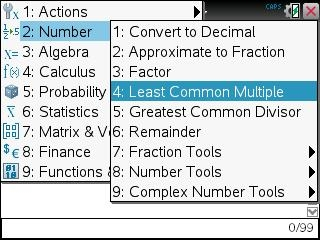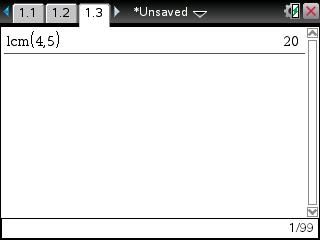# Knowledge Base

## Solution 33051: Calculating lcm() (Least Common Multiple) of Two Arguments on the TI-Nspire™ Family Products.

### How do i find the least common multiple of two arguments on the TI-Nspire Family Products?

The lcm() function returns the least common multiple (LCM) of two arguments. The LCM of two fractions is the LCM of their numerators divided by the greatest common divisor (GCD) of their denominators. The LCM of fractional floating-point numbers is their product. For two lists or matrices, lcm() returns the LCMs of the corresponding elements. The proper syntax for the lcm() function are as follows:

lcm(number1,number2) Þ expression
lcm(list1,list2) Þ list
lcm(matrix1,matrix2) Þ matrix

Please Note: If you are computing the LCM of more than two numbers, you will need to compute the LCM of the first two numbers and then use the answer to compute the LCM with the third number, and so on.

The following example demonstrates how to use lcm():

Example:  Find the LCM of 4 and 5.

The lcm() function can be assessed by opening a calculator document page